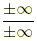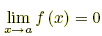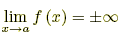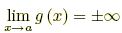index: click on a letter A B C D E F G H I J K L M N O P Q R S T U V W X Y Z A to Z index index: subject areas numbers & symbols sets, logic, proofs geometry algebra trigonometry advanced algebra & pre-calculus calculus advanced topics probability & statistics real world applications multimedia entrieswww.mathwords.com about mathwords website feedback

L'Hôpital's Rule
L'Hospital's Rule

A technique used to evaluate limits of fractions that evaluate to the indeterminate expressionsand. This is done by finding the limit of the derivatives of the numerator and denominator.

Note: Most limits involving other indeterminate expressions can be manipulated into fraction form so that l'Hôpital's rule can be used.

 L'Hôpital's Rule: If f and g are differentiable on an open interval containing a such that g(x) ≠ 0 for all x ≠ a in the interval, and if eitherandorandthenExample:this page updated 19-jul-17 Mathwords: Terms and Formulas from Algebra I to Calculus written, illustrated, and webmastered by Bruce Simmons Copyright © 2000 by Bruce Simmons All rights reserved